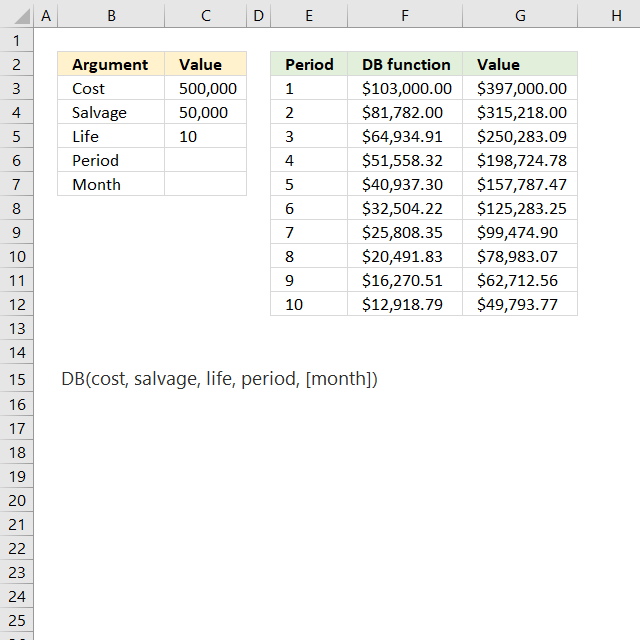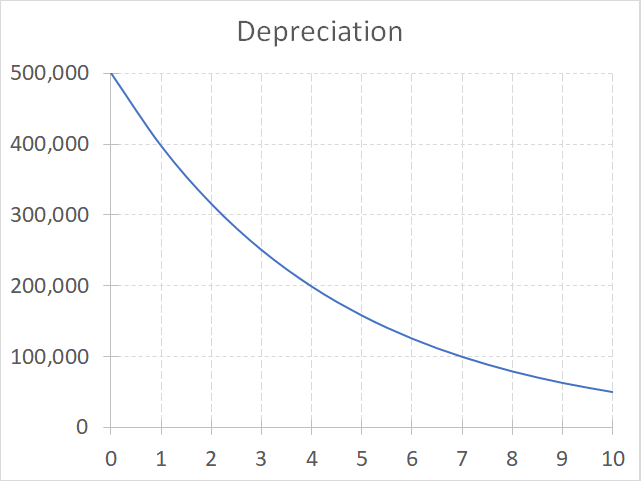Author: Oscar Cronquist Article last updated on September 04, 2018The DB function calculates the depreciation of an asset for a given period using the fixed-declining balance method.

Formula in cell F3:

=DB(\$C\$3,\$C\$4,\$C\$5,E3)

### Excel Function Syntax

DB(cost, salvage, life, period, [month])

### Arguments

 Cost Required. What you pay for the asset. Salvage Required. The value of the asset at the end of depreciation. Life Required. The number of periods the asset is being depreciated. Period Required. The period you want to know the depreciation of. [Month] Optional. The number of months in the first year.

The DB function calculates the depreciation of a period like this:

(cost - total depreciation from prior periods) * rate

where:

rate = 1 - ((salvage / cost) ^ (1 / life))

The first period is calculated like this:

cost * rate * [month] / 12

The last period is calculated like this:

((cost - total depreciation from prior periods) * rate * (12 - [month])) / 12